JEE  >  JEE Main Mock - 8 (Previous Pattern)

# JEE Main Mock - 8 (Previous Pattern)

Test Description

## 90 Questions MCQ Test JEE Main & Advanced Mock Test Series | JEE Main Mock - 8 (Previous Pattern)

JEE Main Mock - 8 (Previous Pattern) for JEE 2023 is part of JEE Main & Advanced Mock Test Series preparation. The JEE Main Mock - 8 (Previous Pattern) questions and answers have been prepared according to the JEE exam syllabus.The JEE Main Mock - 8 (Previous Pattern) MCQs are made for JEE 2023 Exam. Find important definitions, questions, notes, meanings, examples, exercises, MCQs and online tests for JEE Main Mock - 8 (Previous Pattern) below.
Solutions of JEE Main Mock - 8 (Previous Pattern) questions in English are available as part of our JEE Main & Advanced Mock Test Series for JEE & JEE Main Mock - 8 (Previous Pattern) solutions in Hindi for JEE Main & Advanced Mock Test Series course. Download more important topics, notes, lectures and mock test series for JEE Exam by signing up for free. Attempt JEE Main Mock - 8 (Previous Pattern) | 90 questions in 180 minutes | Mock test for JEE preparation | Free important questions MCQ to study JEE Main & Advanced Mock Test Series for JEE Exam | Download free PDF with solutions
 1 Crore+ students have signed up on EduRev. Have you?
JEE Main Mock - 8 (Previous Pattern) - Question 1

### The area bounded by the curves y = sin x between the ordinates x = 0, x = π and the x-axis is

JEE Main Mock - 8 (Previous Pattern) - Question 2

### The 10th term of the expansion of (x-1)11 (in decreasing powers of x) is

JEE Main Mock - 8 (Previous Pattern) - Question 3

### x=7 touches the circle x2 + y2 - 4x - 6y - 12 = 0, then the co-ordinates of the point of contact are

JEE Main Mock - 8 (Previous Pattern) - Question 4

The complex numbers 1,-1,i√3 form a triangle which is

JEE Main Mock - 8 (Previous Pattern) - Question 5

The solution of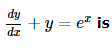Detailed Solution for JEE Main Mock - 8 (Previous Pattern) - Question 5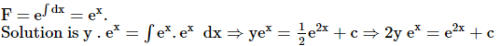JEE Main Mock - 8 (Previous Pattern) - Question 6

The volume of a solid which is obtained by revolving area bounded by an ellipse x2+9y2=9 and straight line x+3y=3 about y-axis is

JEE Main Mock - 8 (Previous Pattern) - Question 7
The solution of the equation x(dy/dx)=y-xtan(y/x) is
JEE Main Mock - 8 (Previous Pattern) - Question 8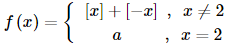where [x] denotes the greatest integer less than or equal to x, is continuous at x = 2, then the value of a is equal to

JEE Main Mock - 8 (Previous Pattern) - Question 9

What is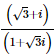equal to?

JEE Main Mock - 8 (Previous Pattern) - Question 10

If x/a + y/b = √2 touches the ellipse (x2/a2) + (y2/b2) =1, then its eccentric angle θ is equal to

JEE Main Mock - 8 (Previous Pattern) - Question 11

If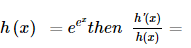JEE Main Mock - 8 (Previous Pattern) - Question 12

If f(4)=4, f'(4) = 3, then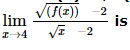Detailed Solution for JEE Main Mock - 8 (Previous Pattern) - Question 12 Apply L'HOPITAL We get {f'(x)/2√f(x)}/{1/2√x} Now put x=4 => (3/2√4)/(1/2√4) => 3
JEE Main Mock - 8 (Previous Pattern) - Question 13

In the following question, a Statement of Assertion (A) is given followed by a corresponding Reason (R) just below it. Read the Statements carefully and mark the correct answer-
Assertion(A): In the binomial expansion of (a-b)n , n ≤ 5, the sum of the 5th and 6th terms is zero, then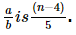Reason(R): In the expansion of (x+y)n, (r+1) th term from the beginning is called the general term Tr+1 Tr+1 = nC, xn-r yr

JEE Main Mock - 8 (Previous Pattern) - Question 14
Negation of "Sohan is in class X or Rani is in class XII" is
JEE Main Mock - 8 (Previous Pattern) - Question 15

In the following question, a Statement of Assertion (A) is given followed by a corresponding Reason (R) just below it. Read the Statements carefully and mark the correct answer-
Assertion(A):The function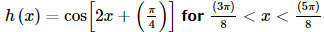is increasing function.
Reason(R): A function f(x) is said to be an increasing function (or monotonically increasing) at an interval a < x < b, if for any two points x1 and x2 of the interval f(x1) < f(x2) when x1 < x2.

JEE Main Mock - 8 (Previous Pattern) - Question 16

In the following question, a Statement of Assertion (A) is given followed by a corresponding Reason (R) just below it. Read the Statements carefully and mark the correct answer-
Assertion(A): A student is allowed to select at the most n books from a collection of (2n+1) books. If the total number of ways in which he can select a book is 63, then n is 3.
Reason(R): By the property of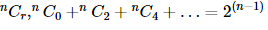JEE Main Mock - 8 (Previous Pattern) - Question 17

In the following question, a Statement of Assertion (A) is given followed by a corresponding Reason (R) just below it. Read the Statements carefully and mark the correct answer-
Assertion(A): Equation of a straight line through the point (3,1,-6) and parallel to each of the two planes 2x - 3y + z + 5 = 0 and x + y + 2z = 2 is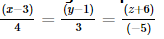Reason(R): The required line will be parallel to the line of intersection of the two planes.

JEE Main Mock - 8 (Previous Pattern) - Question 18
The number of solutions of 2x + y = 4, x - 2 y = 2, 3x + 5y = 6 is
JEE Main Mock - 8 (Previous Pattern) - Question 19
Which of the deciles are less than first quartile?
JEE Main Mock - 8 (Previous Pattern) - Question 20

The pole of the straight line x − 2y + 4 = 0 with respect to the parabola y2 = 6 x is

JEE Main Mock - 8 (Previous Pattern) - Question 21
If P(A∪B)=P(A∩B), then the relation between P(A) and P(B) is
JEE Main Mock - 8 (Previous Pattern) - Question 22

The numbers of all words formed from the letters of the word CALCUTTA is

Detailed Solution for JEE Main Mock - 8 (Previous Pattern) - Question 22

No of letter in the given word is 8
No of repeated word is "C" 2 times, "A" 2 times and "T" 2 times. So the equation is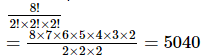JEE Main Mock - 8 (Previous Pattern) - Question 23

Minimum area of the triangle formed by any tangent to the ellipse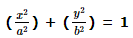with the coordinate axes is

Detailed Solution for JEE Main Mock - 8 (Previous Pattern) - Question 23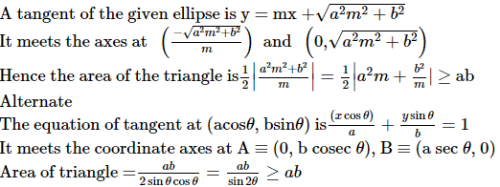JEE Main Mock - 8 (Previous Pattern) - Question 24

If log(a + c), log(c - a), log (a - 2b + c) are in A.P., then

JEE Main Mock - 8 (Previous Pattern) - Question 25
In a binomial distribution, mean is 5 and the variance is 4. Then the number of trials is
JEE Main Mock - 8 (Previous Pattern) - Question 26
The range of the function f (x) = cos [x], where (- π/2) < x < ( π/2), is
JEE Main Mock - 8 (Previous Pattern) - Question 27

The angle between two diagonals of a cube is

JEE Main Mock - 8 (Previous Pattern) - Question 28
The projection of vector 2i+3j-2k on i+2j+3k is
JEE Main Mock - 8 (Previous Pattern) - Question 29
The maximum value of sin θ cos θ is
JEE Main Mock - 8 (Previous Pattern) - Question 30
If dot product of vectors (i+j+k) and the unit vector along the sum of vectors (2i+4j-5k) and (bi+2j+3k) is 1, the value of b is
JEE Main Mock - 8 (Previous Pattern) - Question 31

The correct order of boiling points for primary (1º), secondary (2º) and tertiary (3º) alcohols is

JEE Main Mock - 8 (Previous Pattern) - Question 32

The metal ion which is precipitated when HS is passed with HCl is

JEE Main Mock - 8 (Previous Pattern) - Question 33

The atomic mass of an atom is due to

JEE Main Mock - 8 (Previous Pattern) - Question 34

The most reactive nucleophile among the following is

Detailed Solution for JEE Main Mock - 8 (Previous Pattern) - Question 34

Although due to +I effect of methyl groups, (CH3)2 CHO and (CH3)3 CO are stronger bases but due to steric hindrance both are weaker nucleophiles than CH3O
Further due to resonance, C6H5O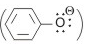is the weakest nucleophile
CH3O is most reactive (stronger) nucleophile

JEE Main Mock - 8 (Previous Pattern) - Question 35

Scurvy is caused due to deficiency of

JEE Main Mock - 8 (Previous Pattern) - Question 36

The molecule which has zero dipole moment is

JEE Main Mock - 8 (Previous Pattern) - Question 37

Which of the following species is paramagnetic?

JEE Main Mock - 8 (Previous Pattern) - Question 38

Which of the following will have the shape of trigonal bipyramid?

Detailed Solution for JEE Main Mock - 8 (Previous Pattern) - Question 38

PF3Cl2 has triogonal bipyramidal geometry. IF5, BrF5 and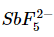have octahedral geometries with one position occupied by a lone pair of electrons.

JEE Main Mock - 8 (Previous Pattern) - Question 39

A reaction is not feasible ,if

JEE Main Mock - 8 (Previous Pattern) - Question 40

The equilibrium between water and its vapour, in an open-vessel

Detailed Solution for JEE Main Mock - 8 (Previous Pattern) - Question 40

In an open vessel, the water vapour diffuse into air and therefore, the concentration of water vapour above water surface is negligible.
The reverse process of condensation of vapour into liquid form is almost absent.
Therefore, evaporation process continues, till the entire water changes into vapour form.
Therefore, in an open vessel, the water vapour system is never in equilibrium.
Finally the whole water will diffuse into air as water vapour.

JEE Main Mock - 8 (Previous Pattern) - Question 41

The heat of combustion of graphite and carbon monoxide respectively are -393.5 kJ mol⁻1 and -283 kJ mol⁻1. Thus, heat of formation of carbon monoxide in kJ mol⁻1 is

JEE Main Mock - 8 (Previous Pattern) - Question 42

The rate of a reaction can be increased in general by all the factors except by

JEE Main Mock - 8 (Previous Pattern) - Question 43

A Carnot engine has an efficiency of 20%. If energy is fed into the engine at the rate of 1 kW, then the output of the engine is

JEE Main Mock - 8 (Previous Pattern) - Question 44

Given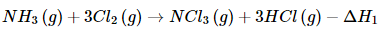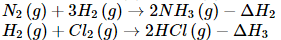The heat of formation of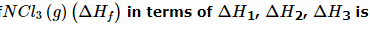JEE Main Mock - 8 (Previous Pattern) - Question 45

Which of the following IUPAC names is correct?

JEE Main Mock - 8 (Previous Pattern) - Question 46

Eka-aluminium and eka-silicon are known as

Detailed Solution for JEE Main Mock - 8 (Previous Pattern) - Question 46

Gallium and Germanium were unknown at the time when Mendeleev's periodic table was formulated. But Mendeleev's predicted the properties of these elements and named them eka-aluminium and eka-silicon. Later when these elements were discovered, their properties were found to be similar to those of aluminium and silicon.

JEE Main Mock - 8 (Previous Pattern) - Question 47

Which of the following system has maximum number of unpaired electrons?

Detailed Solution for JEE Main Mock - 8 (Previous Pattern) - Question 47

Distribution of d-electrons in weak field or high spin complex in tetrahedral field for d6 ion is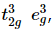thus there are four unpaired electrons.
d9 (octahedral) =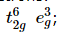no of unparied electron is one
d4 (octahedral, low spin)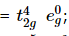no of unpaired electron is one
d7 (octahedral, high spin)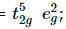no of unparied electron is three.

JEE Main Mock - 8 (Previous Pattern) - Question 48

Which of the following has highest first ionization potential?

Detailed Solution for JEE Main Mock - 8 (Previous Pattern) - Question 48

Nitrogen has highest first ionization potential. This is because N contains exactly half-filled p-orbitals which is extra stable. hence its first ionisation potential is high.
Electronic configuration of N: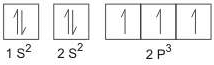JEE Main Mock - 8 (Previous Pattern) - Question 49

The tendency to form complex compounds is maximum for

JEE Main Mock - 8 (Previous Pattern) - Question 50

Which is the best description of the behaviour of bromine in the reaction: H₂O + Br₂ → HOBr + HBr?

JEE Main Mock - 8 (Previous Pattern) - Question 51

Conductivity (Unit:siemen's S) is directly proportionally to the area of the vessel and the concentration of the solution in it and is inversely proportional to the length of vessel,then the unit of constant of proportionally is

JEE Main Mock - 8 (Previous Pattern) - Question 52

What is the temperature of hydrogen gas, which has the same r.m.s. velocity as that of oxygen at a temperature of 0ºC?

JEE Main Mock - 8 (Previous Pattern) - Question 53

NH₃ has a much higher b.p. than PH₃ because

JEE Main Mock - 8 (Previous Pattern) - Question 54

If an isotope of hydrogen has two neutrons in its atoms,its atomic number and atomic mass number will respectively be

JEE Main Mock - 8 (Previous Pattern) - Question 55

Which of the following compounds will be most easily attacked by an electrophile ?

JEE Main Mock - 8 (Previous Pattern) - Question 56

Calcium acetate on heating results in

JEE Main Mock - 8 (Previous Pattern) - Question 57

JEE Main Mock - 8 (Previous Pattern) - Question 58

CH2═CHCH3

JEE Main Mock - 8 (Previous Pattern) - Question 59

What amount of bromine will be required to convert 2 g of phenol into 2, 4, 6-Tribromophenol?

JEE Main Mock - 8 (Previous Pattern) - Question 60

Consider the following statement:
"In a sample of butane at room temperature, none of the molecules will be in the least stable conformation".

Detailed Solution for JEE Main Mock - 8 (Previous Pattern) - Question 60

The conformations of butane differ in their relative stabiliities. The anti-periplanar has minimum repulsion between the groups attached tetrahedrally to the two carbon atoms. In synclinal (gauche) conformations, methyl groups are so close that they repel each other, as a result is has 3.8 kJmol-1 more energy than anti conformation. similarly, syn-periplanar is least stable having energy 18.4 kJ mol−1 than anti conformation. Thus, at room temperature, the more stable ones will have larger populations.

JEE Main Mock - 8 (Previous Pattern) - Question 61

The ratio of minimum to maximum wavelength in Balmer series is

Detailed Solution for JEE Main Mock - 8 (Previous Pattern) - Question 61

From Bohr's theory for hydrogen atom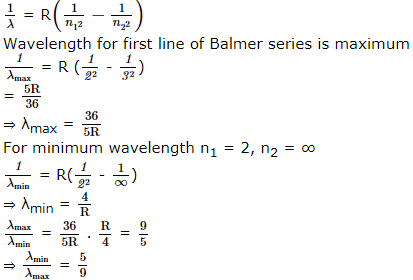JEE Main Mock - 8 (Previous Pattern) - Question 62

In which of the following systems will the radius of the first orbit (n = 1) be minimum?

JEE Main Mock - 8 (Previous Pattern) - Question 63

Identify that set in which all the three sustances are good conductors of electricity

JEE Main Mock - 8 (Previous Pattern) - Question 64

The power of an electric bulb marked as 40 W and 200 V used in a circuit of supply voltage 100 V will be

JEE Main Mock - 8 (Previous Pattern) - Question 65

The curve drawn between velocity and frequency of photon in vacuum will be a

JEE Main Mock - 8 (Previous Pattern) - Question 66

When a charged oil drop moves upwards in an electric field, the electric force acting on the drop is

JEE Main Mock - 8 (Previous Pattern) - Question 67

Out of the folowing statement which is NOT true?

JEE Main Mock - 8 (Previous Pattern) - Question 68

A parallel plate capacitor is first charged and then a dielectric slab is introduced between the plates. The quantity that remains unchanged is

JEE Main Mock - 8 (Previous Pattern) - Question 69

A sheet of aluminium foil of negligible thickness is introduced between the plates of a capacitor. The capacitance of the capacitor

JEE Main Mock - 8 (Previous Pattern) - Question 70

Which of the following statements is true

JEE Main Mock - 8 (Previous Pattern) - Question 71

If radius of the earth contracted 2% and its mass remains the same then weight of the body at the earth surface

JEE Main Mock - 8 (Previous Pattern) - Question 72

On gradually, increasing the temperature of a substance, you will notice which of the following colour?

JEE Main Mock - 8 (Previous Pattern) - Question 73

On a cold morning, a metal surface will feel colder to touch than a wooden surface because

JEE Main Mock - 8 (Previous Pattern) - Question 74

A light string passing over a smooth light pulley connects two blocks of masses m₁ and m₂ (vertically). If the acceleration of the system is (g/8), then the ratio of masses is

JEE Main Mock - 8 (Previous Pattern) - Question 75

The electric current in a circular coil of two turns produced a magnetic induction of 0.2T at its centre. The coil is unwound and is rewound into a circular coil of four turns. The magnetic induction at the centre of the coil now is, in tesla (if same current flows in the coil)

JEE Main Mock - 8 (Previous Pattern) - Question 76

A body thrown with an initial speed of 96 ft/sec reaches the ground after (g=32 ft/sec2)

JEE Main Mock - 8 (Previous Pattern) - Question 77

A simple pendulum of length l has a bob of mass m, with a charge q on it. A vertical sheet of charge, with surface charge density σ passes through the point of suspension. At equilibrium, the spring makes an angle θ with the vertical. its time period oscillations is T in this poisiton. Then

JEE Main Mock - 8 (Previous Pattern) - Question 78

In the following question, a Statement of Assertion (A) is given followed by a corresponding Reason (R) just below it. Read the Statements carefully and mark the correct answer-
Assertion(A): If velocity-time graph is a straight line parallel to time axis, then acceleration of body is zero.
Reason(R): Acceleration is equal to rate of change of velocity (constant).

JEE Main Mock - 8 (Previous Pattern) - Question 79

In the following question, a Statement of Assertion (A) is given followed by a corresponding Reason (R) just below it. Read the Statements carefully and mark the correct answer-
Assertion(A): A ball of mass m fails from a height and bounces back to same height. Contact force is equal to rate of change of momentum.
Reason(R): Contact force is upwards.

JEE Main Mock - 8 (Previous Pattern) - Question 80

In the following question, a Statement of Assertion (A) is given followed by a corresponding Reason (R) just below it. Read the Statements carefully and mark the correct answer-
Assertion(A): Two blocks of masses m1 and m2 are at rest. They are moving towards each other under the mutual internal force. The velocity of centre of mass is zero.
Reason(R): If no external force acts on the system, then velocity of centre of mass is unchanged but can never be zero.

JEE Main Mock - 8 (Previous Pattern) - Question 81

In a plant a sucrose solution of coefficient of viscosity 0.0015 N s/m2 is driven at a velocity of 10⁻2m/s through xylem vessels of radius 2 μ m and length 5 μ m. The hydrostatic pressure difference across the length of xylem vessels, in N/m2 is

JEE Main Mock - 8 (Previous Pattern) - Question 82

When red glass is heated in dark room, it will seem

JEE Main Mock - 8 (Previous Pattern) - Question 83

An object of weight W and density ρ is submerged in a fluid of density ρ₁. Its appearent weight will be

JEE Main Mock - 8 (Previous Pattern) - Question 84

A mass M is moving with a constant velocity along a line parallel to x-axis. Its angular momentum with respect to origin or z-axis is

JEE Main Mock - 8 (Previous Pattern) - Question 85

Amplitude of vibration of any particle in a standing wave produced along a stretched string depends on

JEE Main Mock - 8 (Previous Pattern) - Question 86

A spring of force constant K is cut into two equal parts. Then the force constant of each piece is

JEE Main Mock - 8 (Previous Pattern) - Question 87

Water falls from a height of 210 m. Assuming whole of energy due to fall is converted into heat the rise in temperature of water would be (J = 4.3 Joule/cal)

JEE Main Mock - 8 (Previous Pattern) - Question 88

If the door of a refrigerator is kept open, then which of the following is true

JEE Main Mock - 8 (Previous Pattern) - Question 89

The difference in the lengths of a mean solar day and sidereal day is about

JEE Main Mock - 8 (Previous Pattern) - Question 90

Which one of the following properties of light does not support wave theory of light?

## JEE Main & Advanced Mock Test Series

2 videos|325 docs|189 tests
 Use Code STAYHOME200 and get INR 200 additional OFF Use Coupon Code
Information about JEE Main Mock - 8 (Previous Pattern) Page
In this test you can find the Exam questions for JEE Main Mock - 8 (Previous Pattern) solved & explained in the simplest way possible. Besides giving Questions and answers for JEE Main Mock - 8 (Previous Pattern), EduRev gives you an ample number of Online tests for practice

## JEE Main & Advanced Mock Test Series

2 videos|325 docs|189 tests